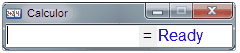# Calculor

A powerful, easy-to-use inline calculator that delivers answers as you type.Features

• Trigonometry functions
• Log, Fabonacci, Factorials
• Variables
• Conversion
• You can even render decimal answers as fractions.  Has memory for recent equations and stored equations.  Even serves as a RUN box (for example, you can type run notepad to open notepad).
• Customizable global hotkey to toggle the window
• You can insert functions from the context menu, or by typing
• Select sections of the equation and push ctrl+enter to calculate the selected part right in the equation (for example, if you have 2+2+3+5 and you select the 2+3 part and push ctrl+enter, the equation turns to 2+5+5)
• Complete Help File
• Version 1.9